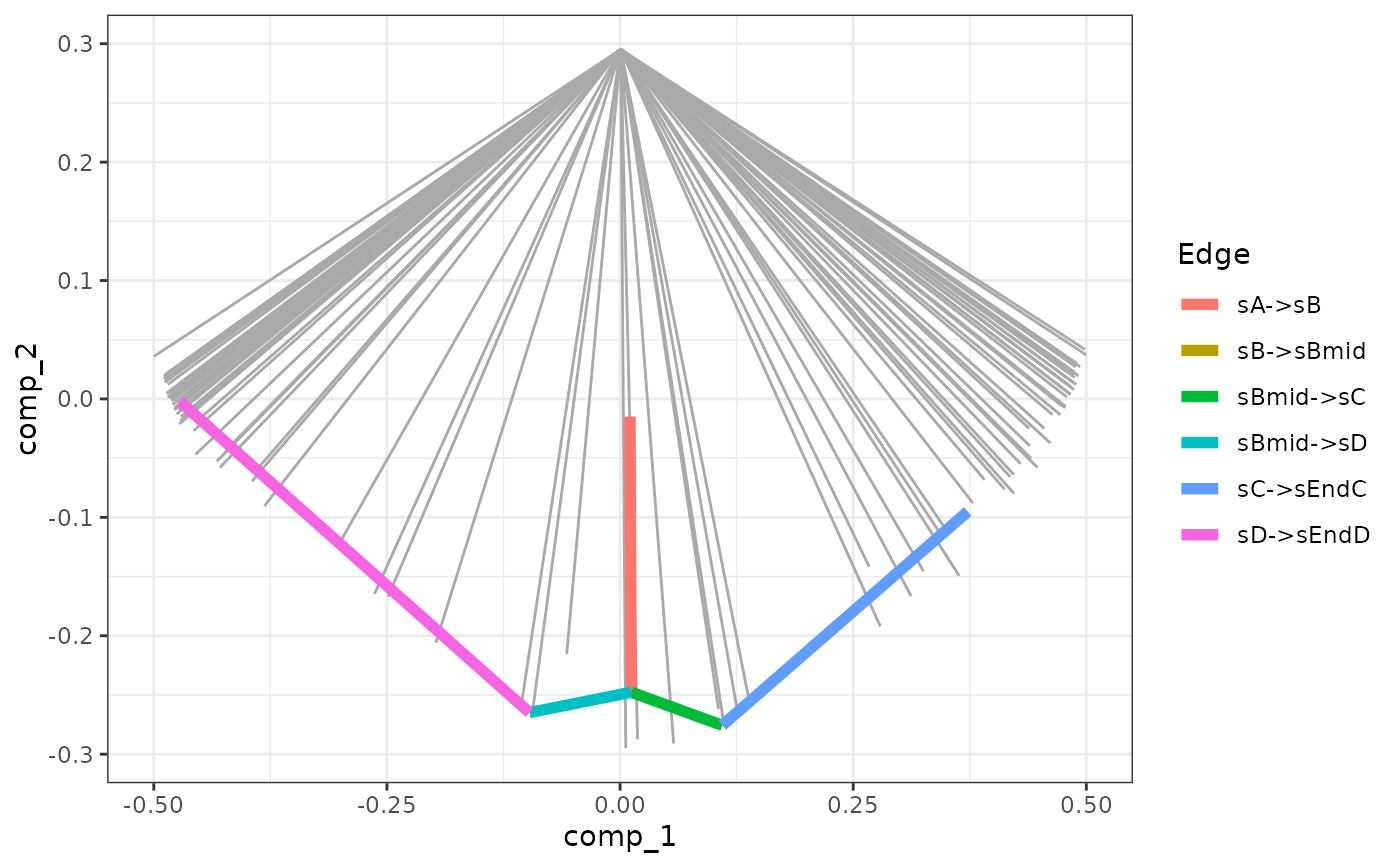Visualise the simulations using the dimred

plot_gold_simulations(
model,
detailed = FALSE,
mapping = aes_string("comp_1", "comp_2"),
highlight = 0
)

## Arguments

model

A dyngen intermediary model for which the simulations have been run with generate_cells().

detailed

Whether or not to colour according to each separate sub-edge in the gold standard.

mapping

Which components to plot.

highlight

Which simulation to highlight. If highlight == 0 then the gold simulation will be highlighted.

## Value

A ggplot2 object.

## Examples

data("example_model")
plot_gold_simulations(example_model)